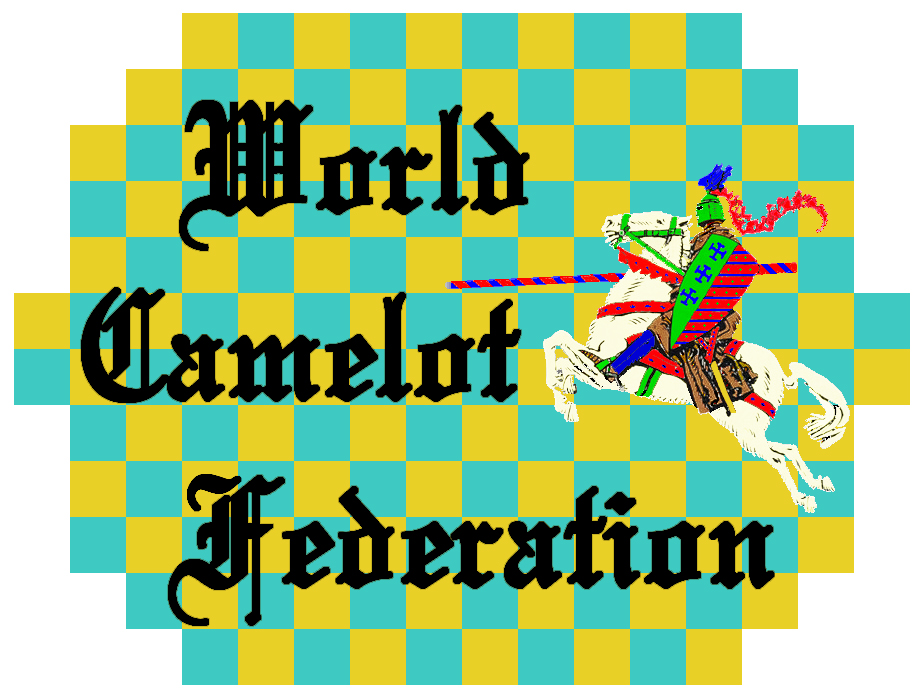In the Camelot middlegame, the trading down of material to reach a winning endgame is of prime importance.  That trade-down action can be quantified in the following manner.

The Knight-Man formula (discussed here) calculates the value of a Knight (N) as:

Let: Man Value = 100

Let: User-defined value of N at start of game = 2.25

P = Total uncastled friendly Pieces

N = 100 + 125 ln(P) / ln(14)

The Trade-Down Bonus formula calculates the value of the bonus (B) as:

Let: D = Difference in Material (White minus Black)

Let: T = Total Material (White plus Black)

B = 1.125 D - .00025 DT

This Bonus, expressed in numerical terms, can then be directly compared to material value.  Following are the Bonus values for all 1,430 combinations of Knights and Men.

(WN = Number of White Knights)

(WM = Number of White Men)

(BN = Number of Black Knights)

(BM = Number of Black Men)